# Math in Focus Grade 6 Chapter 10 Lesson 10.1 Answer Key Area of Triangles

Practice the problems of Math in Focus Grade 6 Workbook Answer Key Chapter 10 Lesson 10.1 Area of Triangles to score better marks in the exam.

## Math in Focus Grade 6 Course 1 B Chapter 10 Lesson 10.1 Answer Key Area of Triangles

### Math in Focus Grade 6 Chapter 10 Lesson 10.1 Guided Practice Answer Key

Hands-On Activity

Materials:

• scissors
• graph paper

PROVE THE FORMULA FOR FINDING THE AREA OF A TRIANGLE

Work in pairs.

Triangle PQR is an acute triangle. $$\overline{\mathrm{Q} R}$$ is the base and PX is the height.

Step 1.
Draw triangle POR on a piece of graph paper as shown. Then draw and label rectangle AQRD,
Example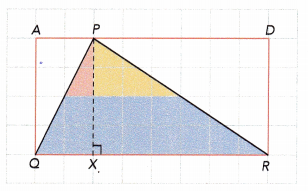Step 2.
Cut up triangle PQR into smaller triangles. Rearrange the triangles to form rectangle EQRF, as shown below.
Example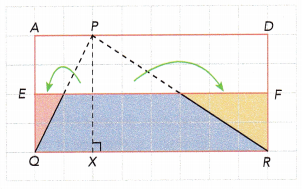The orange, blue, and yellow figures form a rectangle.Step 3.
Find the area of rectangle EQRF. How does its area compare to the area of rectangle AQRD?
Area of rectangle EQRF:
Figure EQRF is occupying 8 units wide and 2 units high.
Area of rectangle = length × width
= 8×2
= 16 sq.units.
Area of rectangle AQRD:
Figure AQRD is occupying 8 units wide and 4 units high.
Area of rectangle = length × width
= 8×4
= 32 sq.units.
By observing the area of EQRF and AQRD, we can say that the area of ANPD is twice the area of AQRD.

How does the area of triangle PQR compare to the area of rectangle EQRF?
Area of triangle MNP:
Let the base of the triangle be PQR , which is occupying 8 horizontal squares. Thus, the base will be 8 units.
The perpendicular segment between the base and the opposite vertex will be the height of the triangle which is 4 units.
Area of triangle = $$\frac{1}{2}$$×b×h
= $$\frac{1}{2}$$×8×4
= $$\frac{1}{2}$$×32
= 16 sq.units
Area of rectangle EQRF:
Figure EQRF is occupying 8 units wide and 2 units high.
Area of rectangle = length × width
= 8×2
= 16 sq.units.
By observing the area of triangle MNP and rectangle ENPF, we can say that both the areas are same.

How does the area of triangle PQR compare to the area of rectangle AQRD?
Area of triangle PQR is 16 sq.units.
Area of rectangle AQRD is 32 sq.units
By observing the area of triangle PQR and the area of rectangle AQRD, we can say that the area of AQRD is twice the area of PQR.

Triangle MNP is an obtuse triangle. $$\overline{N P}$$ is the base and MF is the height.

Step 1.
Draw triangle MNP on a piece of graph paper as shown. Then draw and label rectangle ANPD.
Example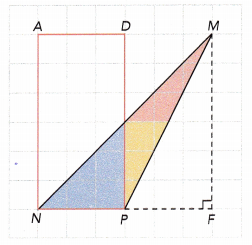Step 2.
Cut up triangle MNP into smaller triangles. Rearrange the triangles to form rectangle ENPF, as shown below.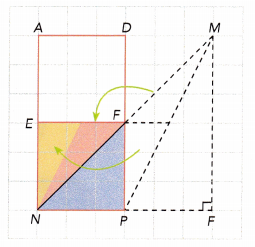Step 3.
Find the area of rectangle ENPF. How does its area compare to the area of rectangle ANPD?
Area of rectangle ENPF:
Figure ENPF is occupying 3 units wide and 3 units high. Since all sides are equal, it is a square.
Area of square = length × length
= 3×3
= 9 sq.units.
Area of rectangle ANPD:
Figure ANPD is occupying 3 units wide and 6 units high, forming a rectangle.
Area of rectangle = length×width
= 3×6
= 18 sq.units
By observing the area of ENPF and ANPD, we can say that the area of ANPD is twice the area of ENPF.

How does the area of triangle MNP compare to the area of rectangle ENPF?
Area of triangle MNP:
Let the base of the triangle be NP, which is occupying 3 horizontal squares. Thus, the base will be 3 units.
The perpendicular segment between the base and the opposite vertex will be the height of the triangle which is 6 units.
Area of triangle = $$\frac{1}{2}$$×b×h
= $$\frac{1}{2}$$×3×6, multiplying 1×3×6 will give 18 and denominator remains same
= $$\frac{18}{2}$$
= 9 sq.units
By observing the area of triangle MNP and rectangle ENPF, we can say that both the areas are same.

How does the area of triangle MNP compare to the area of rectangle ANPD?
Area of triangle MNP is 9 sq.units.
Area of rectangle ANPD is 18 sq.units
By observing the area of triangle MNP and the area of rectangle ANPD, we can say that the area of ANPD is twice the area of MNP.

Complete to find the base, height, and area of each triangle. Each square measures 1 unit by 1 unit.

Question 1.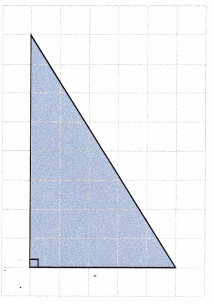Base =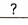units
Height =units
Area = $$\frac{1}{2}$$bh
==units2
Given that:
The above given triangle is drawn on a graph in which each square measures 1 unit.
Let the horizontal side be the base of triangle and the perpendicular segment between the base and the opposite vertex will be the height of the triangle.
The triangle drawn is occupying 5 horizontal square boxes which is base and 8 vertical square boxes which is height.
Thus the base length of the triangle will be 5 units and height will be 8 units.
Base = 5 units, Height = 8 units
Formula:
Area of triangle = $$\frac{1}{2}$$×b×h
= $$\frac{1}{2}$$×5×8, In numerator, multiplying 1×5×8 will give 40 and denominator remains same
= $$\frac{40}{2}$$, 40 divided by 2 gives 20
Therefore, area of the given triangle will be 20 sq.units

Question 2.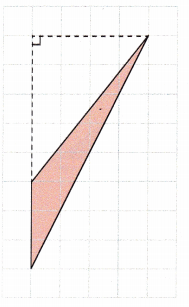Base =units
Height =units
Area = $$\frac{1}{2}$$bh
==units2
The above given triangle is drawn on a graph in which each square measures 1 unit.
Let the vertical side be the base of triangle and the perpendicular segment between the base and the opposite vertex will be the height of the triangle which lies on the horizontal axis.
The vertical boxes are occupying 3 square boxes and horizontally they are occupying 4 boxes.
Thus the base length of the triangle will be 3 units and height will be 4 units.
Base = 3 units, Height = 4 units
Formula:
Area of triangle = $$\frac{1}{2}$$×b×h
= $$\frac{1}{2}$$×3×4, In numerator, multiplying 1×3×4 will give 12 and denominator remains same
= $$\frac{12}{2}$$, 12 divided by 2 gives 6
Therefore, area of the given triangle will be 6 sq.units

Question 3.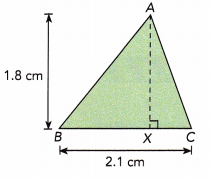Base =cm
Height =cm
Area of triangle ABC
= $$\frac{1}{2}$$bh
==cm2
From the given figure, the height of the triangle is 1.8 cm and base length is 2.1 cm.
Base = 2.1cm
Height = 1.8cm
Area of triangle ABC = $$\frac{1}{2}$$×b×h
= $$\frac{1}{2}$$×2.1×1.8, multiplying 1×2.1×1.8 gives 3.78
= $$\frac{3.78}{2}$$
= 1.89 sq.cm

Question 4.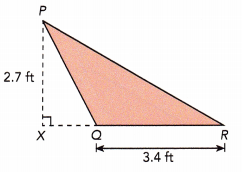Base =ft
Height =ft
Area of triangle PQR
= $$\frac{1}{2}$$bh
==ft2
From the given figure, the height of the triangle is 2.7 ft and base length is 3.4 ft.
Base = 2.7 ft
Height = 3.4 ft
Area of triangle PQR
= $$\frac{1}{2}$$×b×h
= $$\frac{1}{2}$$×3.4×2.7
= $$\frac{1}{2}$$×9.18
= 4.59 sq.ft

Complete to find the height of triangle JKL.

Question 5.
The area of triangle JKL is 35 square meters. Find the height of triangle JKL.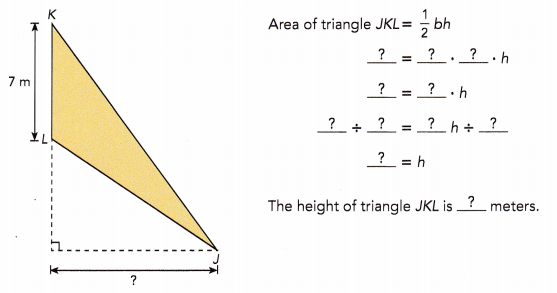Given that the area of triangle JKL is 35 sq.m. The base of the triangle is 7m.
Area of triangle JKL = $$\frac{1}{2}$$×b×h
35 = $$\frac{1}{2}$$×b×h
35 = 0.5×7×h
35 = 3.5×h
35÷3.5 = (3.5×h)÷3.5
10 = h
The height of triangle JKL is 10 m.

Complete to find the base of each triangle.

Question 6.
The area of triangle LMN is 36 square inches. Find the base of triangle LMN.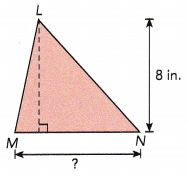Area of triangle LMN = $$\frac{1}{2}$$bh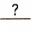=∙ b ∙=∙ b=∙ b÷=b ÷= b
The base of triangle LMN isinches.
Given that the area of triangle LMN is 36 sq.in and the height of the triangle is 8 in.
36 = $$\frac{1}{2}$$×b×h
36 = $$\frac{1}{2}$$×b×8
36 = 4 × b
36 ÷ 4 = (4 ×b) ÷ 4
9 = b
The base of triangle LMN is 9 inches.

Question 7.
The area of triangle PQR1s 19.2 square centimeters. Find the base of triangle PQR.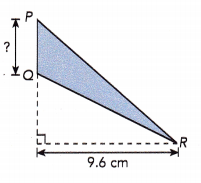Area of triangle PQR = $$\frac{1}{2}$$bh=∙ b ∙=∙ b=∙ b÷=b ÷= b
The base of triangle PQR isinches.
Given that the area of triangle PQR is 19.2 sq.cm and the height of the triangle is 9.6 cm.
Area of triangle PQR = $$\frac{1}{2}$$×b×h
19.2 = $$\frac{1}{2}$$×b×9.6
19.2 = 4.8 × b
19.2÷4.8 = (4.8×b)÷4.8
4 = b
The base of triangle PQR is 4 inches.

### Math in Focus Course 1B Practice 10.1 Answer Key

Identify a base and a height of each triangle.

Question 1.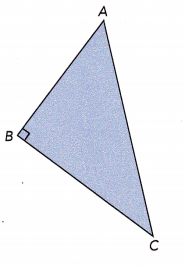Let the side BC be the base of triangle and the perpendicular segment between the base and the opposite vertex will be the height of the triangle,which is AB.
Therefore, the base of triangle is BC and height is AB.

Question 2.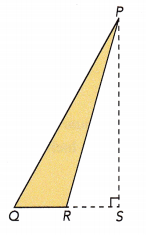Let the horizontal side QR be the base of triangle and the perpendicular segment between the base and the opposite vertex will be the height of the triangle,which is PR.
Therefore, the base of triangle is QR and height is PR.

Copy each triangle. Label a base with the letter b and a height with the letter h.

Question 3.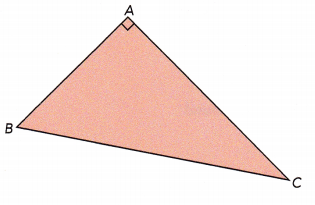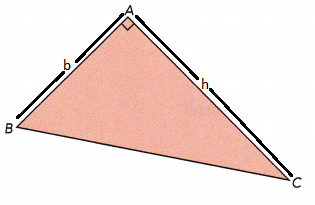Explanation:
Let the side AB be the base of triangle and the perpendicular segment between the base and the opposite vertex will be the height of the triangle,which is AC.
Therefore, the side AB can be marked as b and the side AC as h.

Question 4.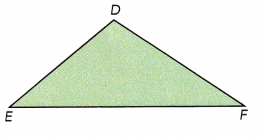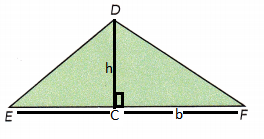Explanation:
Let the side EF be the base of triangle and a perpendicular segment is drawn between the base and the opposite vertex which will be the height of the triangle,which is DC.
Therefore, the side EF can be marked as b and the side DC as h.

Question 5.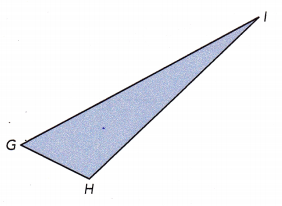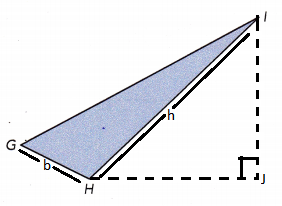Explanation:
Let the side GH be the base of triangle and the perpendicular segment between the base and the opposite vertex will be the height of the triangle,which is HI.
Therefore, the side GH can be marked as b and the side HI as h.

Question 6.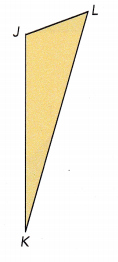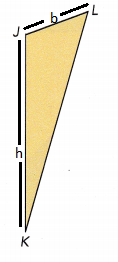Explanation:
Let the side JK be the base of triangle and the perpendicular segment between the base and the opposite vertex will be the height of the triangle,which is JL.
Therefore, the side JK can be marked as b and the side JL as h.

Find the area of each triangle.

Question 7.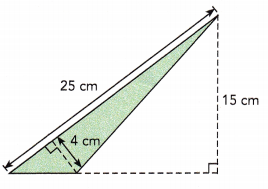Let us assume the side measuring 25 cm be the base of the triangle and the perpendicular segment between the base and the opposite vertex will be the height of the triangle which measures 4 cm.
Thus, the base of triangle = 25 cm
and the height of the triangle = 4 cm
Area of triangle = $$\frac{1}{2}$$×b×h
= $$\frac{1}{2}$$×25×4
= $$\frac{1}{2}$$×100
= 100÷2
= 50
Therefore, the area of the triangle will be 50 sq.cm

Question 8.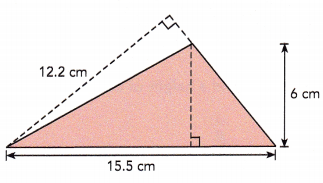Let us assume the horizontal side measuring 15.5 cm be the base of the triangle and the perpendicular segment between the base and the opposite vertex will be the height of the triangle which measures 6 cm.
Thus, the base of triangle = 15.5 cm
and the height of the triangle = 6 cm
Area of triangle = $$\frac{1}{2}$$×b×h
= $$\frac{1}{2}$$×15.5×6
= $$\frac{1}{2}$$×93
= 93÷2
= 46.5
Therefore, the area of the triangle will be 46.5 sq.cm

The area of each triangle is 76 square inches. Find the height and round your answer to the nearest tenth of an inch.

Question 9.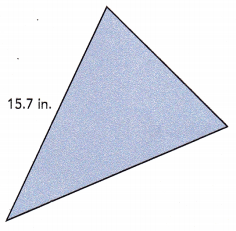Given that the area of triangle is 76 sq.in and base is 15.7 in
We now that, Area of triangle = $$\frac{1}{2}$$×b×h
76 = $$\frac{1}{2}$$×15.7×h
76 = (15.7÷2)×h
76 = 7.85 × h
76 ÷ 7.85 = (7.85 × h)÷7.85
9.6 = h
The value nearest to tenth will be 10 in.
Therefore, the height of the given triangle will be 10 in.

Question 10.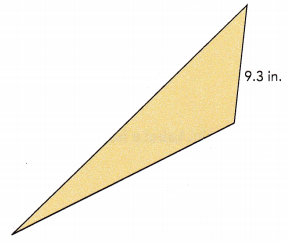Given that the area of triangle is 76 sq.in and base is 9.3 in
We now that, Area of triangle = $$\frac{1}{2}$$×b×h
76 = $$\frac{1}{2}$$×9.3×h
76 = (9.3÷2)×h
76 = 4.65 × h
76 ÷ 4.65 = (4.65×h)÷4.65
16.3 = h
The value nearest to tenth will be 16 in.
Therefore, the height of the given triangle will be 16 in.

The area of each triangle is 45 square centimeters. Find the base and round your answer to the nearest tenth of a centimeter if necessary.

Question 11.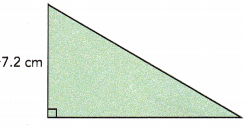Given that the area of triangle is 45 sq.cm and height is 7.2 cm
We now that, Area of triangle = $$\frac{1}{2}$$×b×h
45 = $$\frac{1}{2}$$×b×7.2
45 = (7.2 ÷ 2)×b
45 = 3.6 × b
45 ÷ 3.6 = (3.6 × b)÷3.6
12.5 = b
The value nearest to tenth will be 12 in.
Therefore, the base of the given triangle will be 12 in.

Question 12.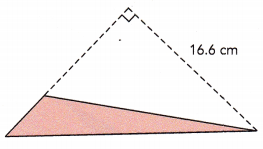Given that the area of triangle is 45 sq.cm and height is 16.6 cm
We now that, Area of triangle = $$\frac{1}{2}$$×b×h
45 = $$\frac{1}{2}$$×b×16.6
45 = 8.3 × b
45 ÷ 8.3 = (8.3 × b) ÷ 8.3
5.4 = b
The value nearest to base will be 5 cm.
Therefore, the base of the given triangle will be 5 cm.

Question 13.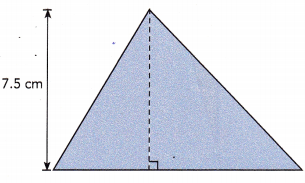Given that the area of triangle is 45 sq.cm and height is 7.5 cm
We now that, Area of triangle = $$\frac{1}{2}$$×b×h
45 = $$\frac{1}{2}$$×b×7.5
45 = 3.75 × b
45 ÷ 3.75 = (3.75 × b)÷ 3.75
12 = b
Therefore, the base of the given triangle will be 12 cm.

Question 14.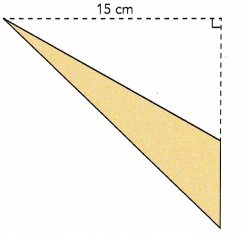Given that the area of triangle is 45 sq.cm and height is 15 cm
We now that, Area of triangle = $$\frac{1}{2}$$×b×h
45 = $$\frac{1}{2}$$×b×15
45 = 7.5 × b
45 ÷ 7.5 = (7.5×b) ÷ 7.5
6 = b
Therefore, the base of the given triangle will be 6 cm.

Find the area of the shaded region.

Question 15.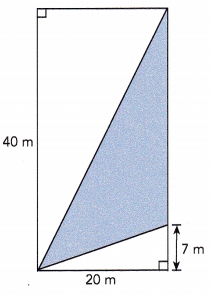Total Area:
Given rectangle measures 20m wide and 40m long.
Area of rectangle = length×width
= 20×40
= 800 sq.m
Larger triangle:
Let us assume the 40 m line segment as base and the perpendicular segment between the base and the opposite vertex will be the height of the triangle which measures 20 m.
Area of larger triangle = $$\frac{1}{2}$$×b×h
= $$\frac{1}{2}$$×40×20
= $$\frac{1}{2}$$×800
= 800÷2
= 400 sq.m
Smaller triangle:
Let us assume the 20 m line segment as base and the perpendicular segment between the base and the opposite vertex will be the height of the triangle which measures 7 m.
Area of larger triangle = $$\frac{1}{2}$$×b×h
= $$\frac{1}{2}$$×20×7
= $$\frac{1}{2}$$×140
= 140÷2
= 70 sq.m
Area of shaded region = Total Area – (Area of larger triangle + Area of smaller triangle)
= 800 – (400+70)
= 800 – 470
= 330 sq.m

Question 16.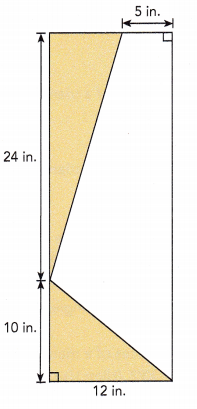Larger triangle:
Let us assume the 24 in line segment as base and the perpendicular segment between the base and the opposite vertex will be the height of the triangle which measures (12-5)=7 in.
Area of larger triangle = $$\frac{1}{2}$$×b×h
= $$\frac{1}{2}$$×24×7
= $$\frac{1}{2}$$×168
= 168÷2
= 84 sq.in
Smaller triangle:
Let us assume the 12 in line segment as base and the perpendicular segment between the base and the opposite vertex will be the height of the triangle which measures 10 in.
Area of larger triangle = $$\frac{1}{2}$$×b×h
= $$\frac{1}{2}$$×12×10
= $$\frac{1}{2}$$×120
= 120÷2
= 60 sq.m
Area of shaded region = Area of larger triangle + Area of smaller triangle
= 84 + 60
= 144 sq.in

Question 17.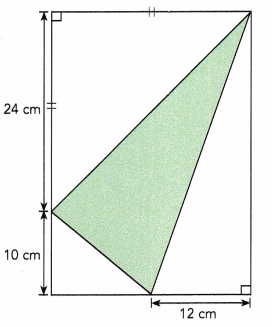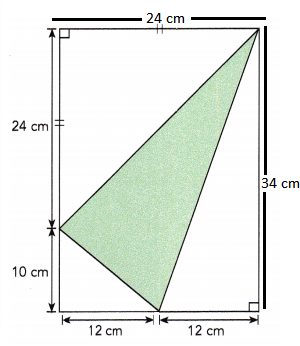First triangle:
Let us assume the base of first triangle be 12 cm and the perpendicular segment between the base and the opposite vertex will be the height of the triangle which measures 34 cm.
Area of first triangle = $$\frac{1}{2}$$×b×h
= $$\frac{1}{2}$$×12×34
= $$\frac{1}{2}$$×408
= 408÷2
= 204 sq.cm
Second triangle:
Let us assume the base of second triangle be 12 cm and the perpendicular segment between the base and the opposite vertex will be the height of the triangle which measures 10 cm.
Area of second triangle = $$\frac{1}{2}$$×b×h
= $$\frac{1}{2}$$×12×10
= $$\frac{1}{2}$$×120
= 120÷2
= 60 sq.cm
Third triangle:
Let us assume the base of first triangle be 24 cm and the perpendicular segment between the base and the opposite vertex will be the height of the triangle which measures 24 cm.
Area of first triangle = $$\frac{1}{2}$$×b×h
= $$\frac{1}{2}$$×24×24
= $$\frac{1}{2}$$×576
= 576÷2
= 288 sq.cm
Total Area:
The rectangle measures 24 cm wide and 34 cm high
Area of rectangle = length×width
= 24×34
= 816 sq.cm
Area of shaded region will be =  Total Area – (Area of first triangle + Area of second triangle + Area of third trinagle)
= 816 – (204+60+288)
= 816 – 552
= 264 sq.cm

Question 18.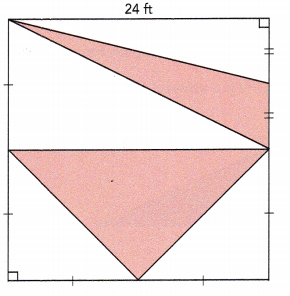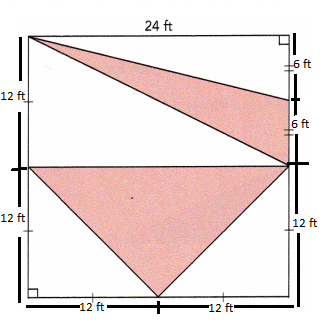Let us assume the base of first triangle be 6 ft and the perpendicular segment between the base and the opposite vertex will be the height of the triangle which measures 24 ft.
Area of first shaded triangle = $$\frac{1}{2}$$×b×h
= $$\frac{1}{2}$$×6×24
= 144÷2
= 72 sq.ft
Area of second shaded triangle = $$\frac{1}{2}$$×b×h
= $$\frac{1}{2}$$×24×12
= 288÷2
= 144 sq.ft
Area of shaded region = Area of first triangle + Area of second triangle
= 72+144
= 216 sq.ft

Question 19.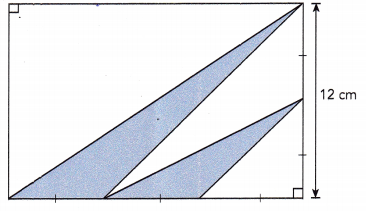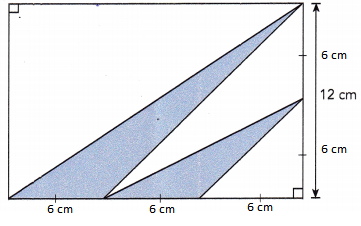Area of larger triangle:
Let us assume the base of first triangle be 6 cm and the perpendicular segment between the base and the opposite vertex will be the height of the triangle which measures 12 cm.
Area of larger triangle = $$\frac{1}{2}$$×b×h
= $$\frac{1}{2}$$×6×12
= 72÷2
= 36 sq.cm
Area of smaller triangle:
Let us assume the base of first triangle be 6 cm and the perpendicular segment between the base and the opposite vertex will be the height of the triangle which measures 6 cm.
= $$\frac{1}{2}$$×6×6
= 36÷2
= 18 sq.cm
Area of shaded region = Area of larger triangle + Area of smaller triangle
= 36 + 18
= 54 sq.cm

Use graph paper. Solve.

Question 20.
The coordinates of the vertices of a triangle are A (4, 7), B (4, 1), and C (8, 1). Find the area of triangle ABC.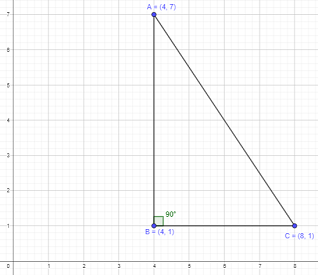Given that the vertices of a triangle are A (4, 7), B (4, 1), and C (8, 1).
Let BC be the base of the triangle and the perpendicular segment between the base and the opposite vertex will be the height of the triangle which is AB.
BC measures 4 units and AB measures 6 units.
Area of triangle ABC = $$\frac{1}{2}$$×b×h
= $$\frac{1}{2}$$×4×6
= 24 ÷ 2
= 12 sq.units

Question 21.
The coordinates of the vertices of a triangle are D (1, 7), E (-3, 2), and F (6, 2). Find the area of triangle DEF.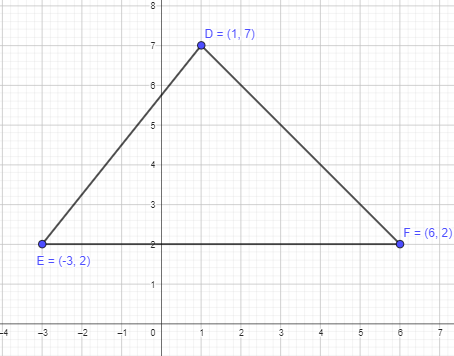Given that the vertices of a triangle are D (1, 7), E (-3, 2), and F (6, 2).
Let EF measuring 9 units be the base of the triangle and the perpendicular segment between the base and the opposite vertex will be the height of the triangle which measures 5 units.
Area of triangle DEF = $$\frac{1}{2}$$×b×h
= $$\frac{1}{2}$$×9×5
= 45 ÷ 2
= 22.5 sq.units

Question 22.
The coordinates of the vertices of a triangle are J (-5, 2), K (1, -2), and L (5, -2). Find the area of triangle JKL.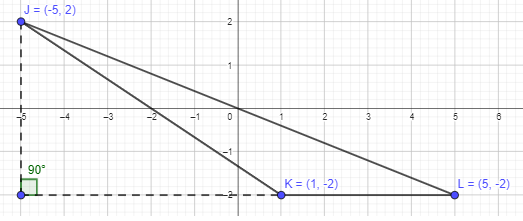Given that the vertices of a triangle are J (-5, 2), K (1, -2), and L (5, -2).
Let LK measuring 4 units be the base of the triangle and the perpendicular segment between the base and the opposite vertex will be the height of the triangle which measures 4 units.
Area of triangle JKL= $$\frac{1}{2}$$×b×h
= $$\frac{1}{2}$$×4×4
= 16 ÷ 2
= 8 sq.units

Question 23.
The area of triangle MNP is 1 7.5 square units. The coordinates of M are (-9, 5), and the coordinates of N are (-2, 0). The height of triangle MNP is 5 units and is perpendicular to the x-axis. Point P lies to the right of point N. Given that $$\overline{N P}$$ is the base of the triangle, find the coordinates of point P.
Given that the area of triangle MNP is 17.5 square units and height of the triangle is 5 units.
Area of triangle = $$\frac{1}{2}$$×b×h
17.5 = $$\frac{1}{2}$$×b×5
17.5 = 2.5×b
(17.5÷2.5) = (2.5xb)÷2.5
7 = b
Thus, the base of the triangle will be 7 units.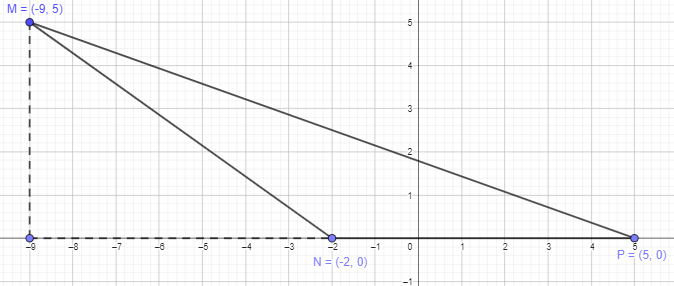Explanation:
From the above calculations, the base of the triangle measures 7 units and lies on the right of the point N.
Move 7 units to the right from the point N and mark the point. The marked point is the required P point.
Thus, the coordinates of P will be (5,0)

Question 24.
The coordinates of the vertices of a triangle are X (1, 2), Y (-6, -2), and Z (1, -4). Find the area of triangle XYZ. (Hint: Use the vertical side as the base.)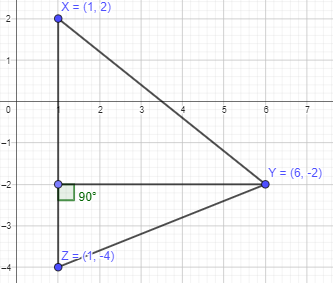Given that the vertices of a triangle are X (1, 2), Y (-6, -2), and Z (1, -4).
As given the base of the triangle is the vertical side, XZ which measures 6 units.
The perpendicular segment between the base and the opposite vertex will be the height of the triangle which measures 5 units.
Area of triangle = $$\frac{1}{2}$$×b×h
= $$\frac{1}{2}$$×6×5
= $$\frac{1}{2}$$×30
= 15 sq.units

Question 25.
The coordinates of the vertices of a triangle are P (-2, 6), Q (-4, 2), and R (5, 1). Find the area of triangle PQR. (Hint: Draw a rectangle around triangle PQR.)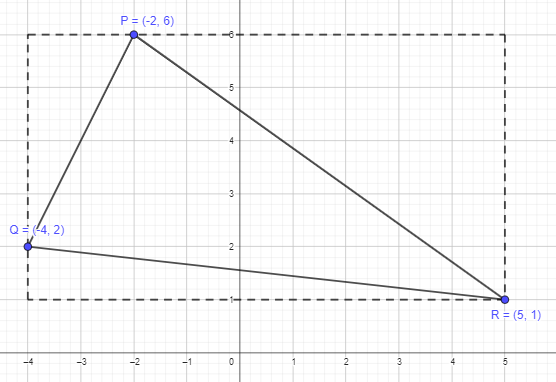Part 1:
From the given figure, we can observe that the rectangle around triangle PQR measures 5 units long and 9 units wide.
Area of rectangle = length× width
= 5×9
= 45 sq.units
The total area of the figure is 45 sq.units
Part 2:
Area of first triangle:
Let us assume the base of first triangle be 2 units and the perpendicular segment between the base and the opposite vertex will be the height of the triangle which measures 4 units
Area of first triangle = $$\frac{1}{2}$$×b×h
= $$\frac{1}{2}$$×2×4
= $$\frac{1}{2}$$×8
= 8÷2
= 4 sq.units
Part 3:
Area of second triangle:
Let us assume the base of second triangle be 7 units and the perpendicular segment between the base and the opposite vertex will be the height of the triangle which measures 5 units
Area of first triangle = $$\frac{1}{2}$$×b×h
= $$\frac{1}{2}$$×7×5
= $$\frac{1}{2}$$×35
= 35÷2
= 17.5 sq.units
Part 4:
Area of third triangle:
Let us assume the base of third triangle be 9 units and the perpendicular segment between the base and the opposite vertex will be the height of the triangle which measures 1 units
Area of first triangle = $$\frac{1}{2}$$×b×h
= $$\frac{1}{2}$$×9×1
= 9÷2
= 4.5 sq.units
Part 5:
Area of the triangle = Total Area-(Area of first triangle+Area of second triangle+Area of third triangle)
= 45-(4+17.5+4.5)
= 45-26
= 19 sq.units

Find the area of the shaded region for questions 26 to 29.

Question 26.
Figure DGHJ is a trapezoid.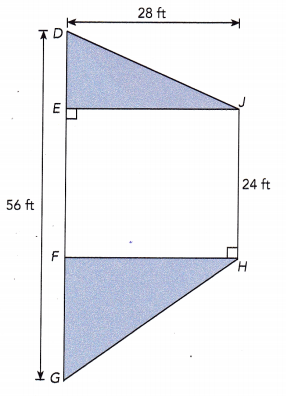Given that figure DGHJ is a trapezoid measuring 28 ft high and two parallel sides with 24ft and 56 ft long.
Area of trapezoid = $$\frac{1}{2}$$×(a+b)×h
= $$\frac{1}{2}$$×(24+56)×28
= $$\frac{1}{2}$$×80×28
= 2240÷2
= 1120 sq.ft
The unshaded figure seems to be square as each angle is 90 degrees. Thus, all sides of it will be equal.
Area of square = 24×24
= 576 sq.ft
Area of the shaded region = Total Area – Area of the unshaded region
= 1120-576
= 544 sq.ft

Question 27.
Figure ABCD is a rectangle. The length of $$\overline{Z B}$$ is $$\frac{3}{7}$$ the length of $$\overline{A B}$$.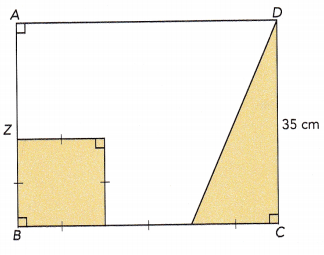Part 1:
From the given figure, we can observe that the area of shaded region will be the sum of areas of square and triangle.
Given that the figure ABCD is a rectangle and the length of $$\overline{Z B}$$ is $$\frac{3}{7}$$ the length of $$\overline{A B}$$.
Length of AB = 35cm
Length of ZB will be $$\frac{3}{7}$$ × AB
= $$\frac{3}{7}$$ × 35
= 3×5
= 15 cm.
Area of square = 15×15
= 225 sq.cm
Area of triangle = $$\frac{1}{2}$$×b×h
= $$\frac{1}{2}$$×15×35
= 525÷2
= 262.5
Part 2:
Total Area of shaded region = Area of sqaure + Area of triangle
= 225 + 262.5
= 487.5

Question 28.
The area of triangle POS is $$\frac{7}{12}$$ of the area of trapezoid PRST.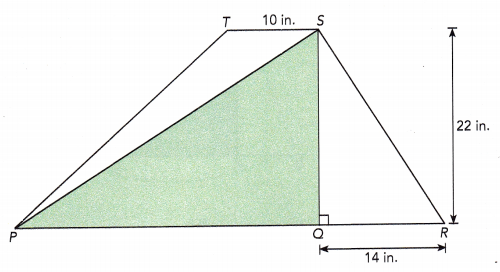Part 1:
Area of first triangle SQR:
Let us assume the base of first triangle be 14 units and the perpendicular segment between the base and the opposite vertex will be the height of the triangle which measures 22 units
Area of first triangle = $$\frac{1}{2}$$×b×h
= $$\frac{1}{2}$$×14×22
= $$\frac{1}{2}$$×308
= 154 sq.in
Part 2:
Area of second triangle PTS:
Let us assume the base of first triangle be 10 units and the perpendicular segment between the base and the opposite vertex will be the height of the triangle which measures 22 units
Area of first triangle = $$\frac{1}{2}$$×b×h
= $$\frac{1}{2}$$×10×22
= $$\frac{1}{2}$$×220
= 110 sq.in
Part 3:
Given that the area of triangle PQS is $$\frac{7}{12}$$ of the area of trapezoid PRST.Let us assue the area of trapezoid as ‘x’ inches.
Then the area of triangle PQS will be $$\frac{7}{12}$$ of the area of trapezoid PRST.
Area of triangle PQS = $$\frac{7}{12}$$ × x
Total Area will be the area of trapezoid.
Total Area = Area of triangle PQS + Area of triangle SQR + Area of triangle PTS
x = ($$\frac{7}{12}$$ × x)+ 154 + 110
x = ($$\frac{7}{12}$$ × x)+ 264
x = $$\frac{7x}{12}$$+ 264
x-$$\frac{7x}{12}$$ = $$\frac{7x}{12}$$–$$\frac{7x}{12}$$+264
($$\frac{12}{12}$$×x)-$$\frac{7x}{12}$$  = 264
($$\frac{12x}{12}$$)-$$\frac{7x}{12}$$ = 264
$$\frac{5x}{12}$$ = 264
$$\frac{5x}{12}$$ × 12 = 264 × 12
5x = 3168
x = 633.6
Thus, the area of trapezoid = 633.6 sq.in
Area of triangle = $$\frac{7}{12}$$ × 633.6
= 4435.2÷12
= 369.6 sq.in

Question 29.
Figure EFHL is a parallelogram. The length of $$\overline{F G}$$ is $$\frac{5}{8}$$ the length of $$\overline{F H}$$.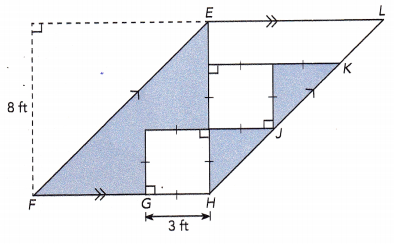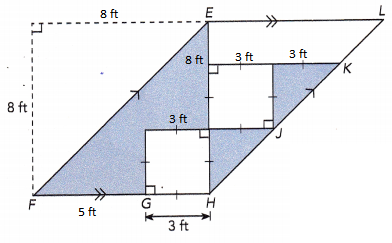Given that:
Part 1:
The length of $$\overline{F G}$$ is $$\frac{5}{8}$$ the length of $$\overline{F H}$$.
Length of FH be ‘x’ ft.Length of FG will be $$\frac{5x}{8}$$
FH length = FG length + GH length
x = $$\frac{5x}{8}$$ + 3
x – $$\frac{5x}{8}$$ = $$\frac{5x}{8}$$–$$\frac{5x}{8}$$+3
($$\frac{8}{8}$$×x)- $$\frac{5x}{8}$$ = $$\frac{5x}{8}$$–$$\frac{5x}{8}$$+3
$$\frac{3x}{8}$$ = 3
$$\frac{3x}{8}$$÷$$\frac{3}{8}$$ = 3÷$$\frac{3}{8}$$
x = 8 ft
Length of FH be 8ft and length of FG will be $$\frac{5}{8}$$ × 8 = 5 units.
In the figure, we can observe two squares, larger square with 8 ft length and smaller square with length of 3 ft.
Area of larger square = 8×8 = 64 sq.ft
Area of smaller square = 3×3 = 9 sq.ft
Figure EFHL is a parallelogram.
Part 2:
Area of first shaded region = Area of larger sqaure – Area of smaller square
= 64-9
= 55 sq.ft
Area of triangle LEH
Let us assume EL as base and EH.
Area of triangle mesauring 3 ft wide and 3 ft high = $$\frac{1}{2}$$×b×h
= $$\frac{1}{2}$$×3×3
= 4.5 sq.ft
There are 2 such triangles = 4.5+4.5 = 9 sq.ft
Part 3:
Area of shaded region will be 55+9 = 64 sq.ft